Author: Oscar Cronquist Article last updated on September 06, 2022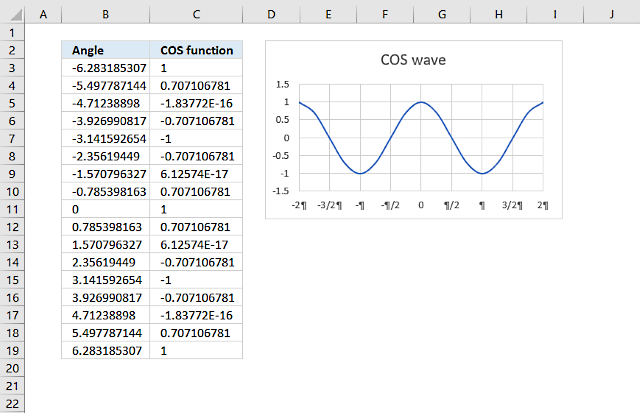The COS function calculates the cosine of an angle.

COS(number)

## 2. COS function Arguments

 number Required. The radian angle you want to know the cosine of.

## 3. COS function example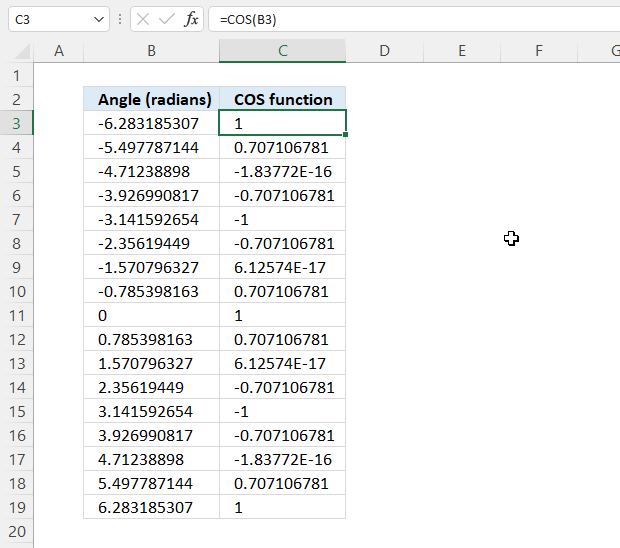Formula in cell C3:

=COS(B3)

## 4. How to calculate cosine using degrees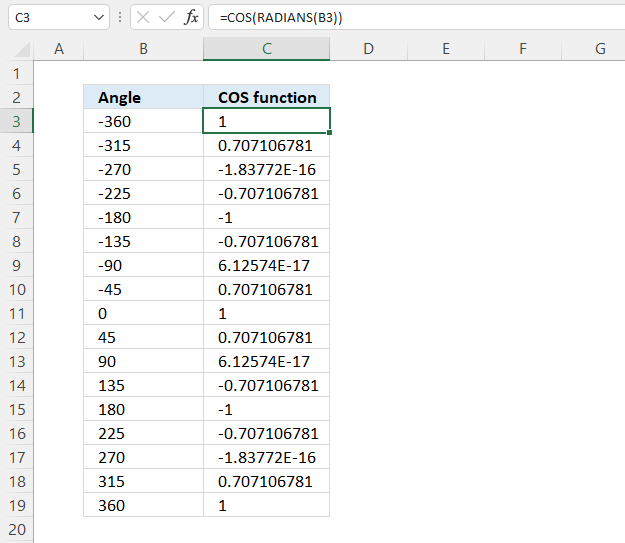Formula in cell C3:

### Explaining formula

#### Step 1 - Convert degrees to radians

becomes

and returns approx. -3.28 or -2*pi.

#### Step 2 - Calculate the cosine

The COS function calculates the cosine of an angle.

Function syntax: COS(number)

becomes

COS(-2*pi)

and returns 0 (zero).

## 5. How to plot a cosine wave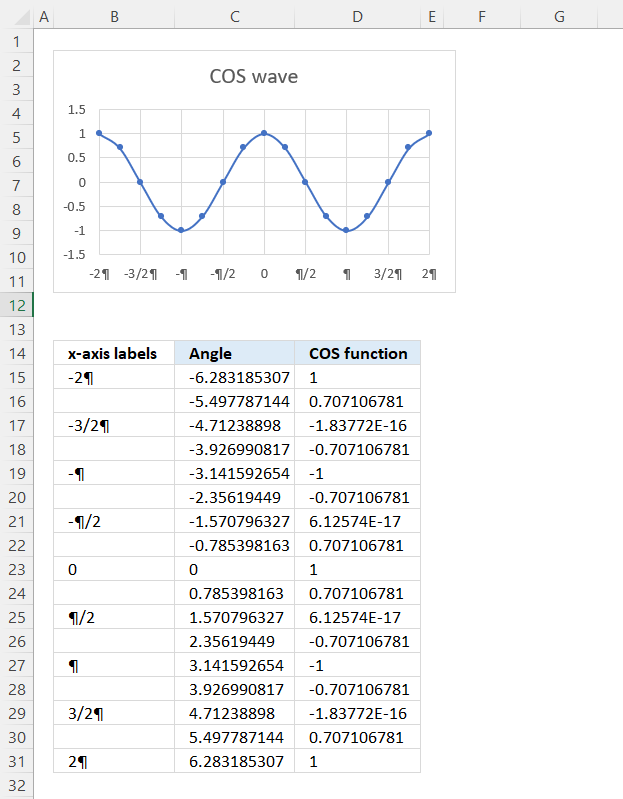The image above demonstrates a scatter chart containing a cosine graph. Here is how to create a cosine plot:

The formula in cell C15 creates radians starting from -2*pi and adds (1/4)*pi for each cell below.

Formula in cell C15:

=-2*PI()+(ROW(A1)-1)*PI()/4

Formula in cell D15:

=COS(C15)

### 5.1 Explaining formula in cell C15

#### Step 1 - Calculate -2*pi

The PI function returns the number pi, it has no arguments.

-2*PI()

becomes

-2*3.14 (approx.)

and returns approx. -6.28

#### Step 2 - Subtract with pi/4

The ROW function returns a number representing the row in a cell reference.
ROW(reference)

A1 is a relative cell reference meaning it changes when the cell is copied to cells below, this creates a sequential list of numbers starting from 1 to n.

(ROW(A1)-1)*PI()/4

becomes

(1-1)*PI()/4

becomes

0*PI()/4

and returns 0 (zero).

#### Step 3 - Calculate radian

-2*PI()+(ROW(A1)-1)*PI()/4

becomes

-6.28 + 0 equals approx. -6.28

### 5.2 How to insert a Scatter chart with Smooth Lines and Markers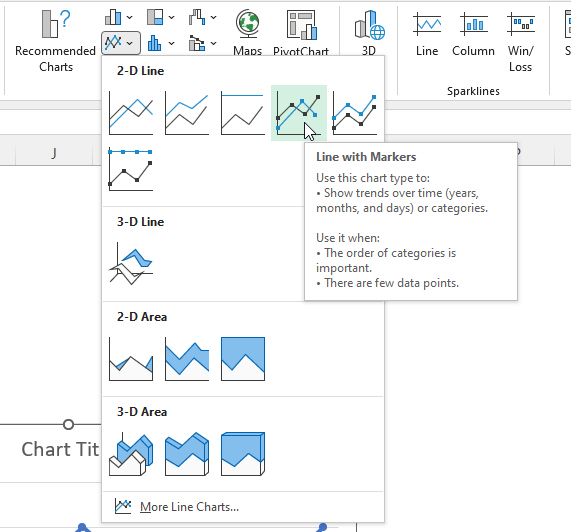1. Select cell range B3:B31.
2. Press and hold CTRL.
3. Select cell range D3:D13.
4. Go to tab "Insert" on the ribbon.
5. Press with left mouse button on the "Insert Line or Area chart" button, and a pop-up menu appears.
6. Press with left mouse button on the "Line with Markers" button, see the image above.
7. A new chart appears on the worksheet, see the image below.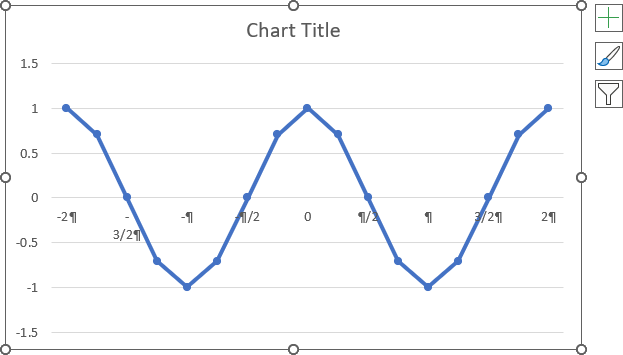Double press with left mouse button on the chart to open the "Format Chart Area Pane".

Select the line on the chart, press with left mouse button on the checkbox "Smoothed Line" on the "Format Chart Area Pane".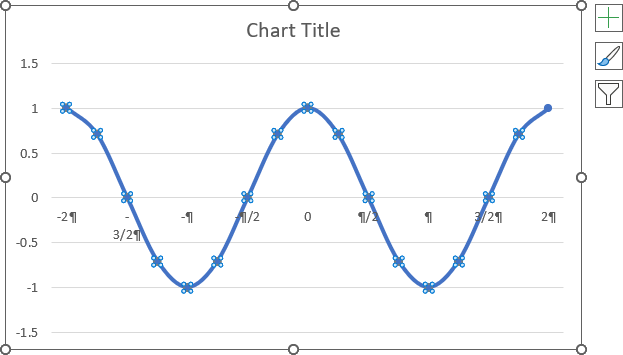Double-press with left mouse button on the y-axis values, the "Format Axis Pane" shows up.

Press with mouse on the radio button "Axis value:" and type -1.5 (Horizontal Axis crosses)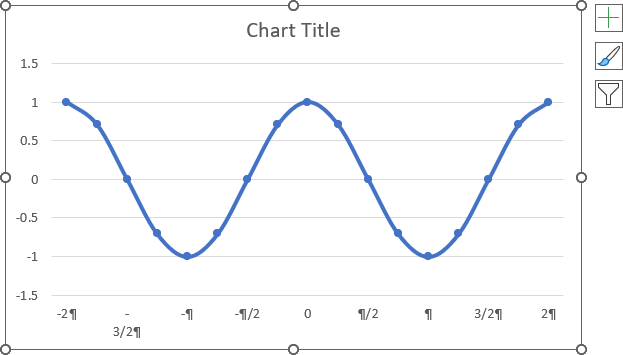Press with right mouse button on on x-axis values. Press with mouse on "Add Major gridlines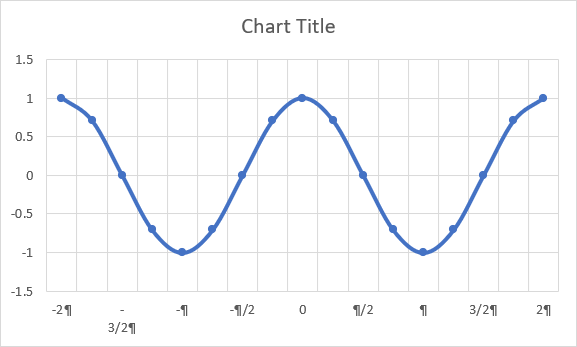## 6. How to change the cosine wave amplitude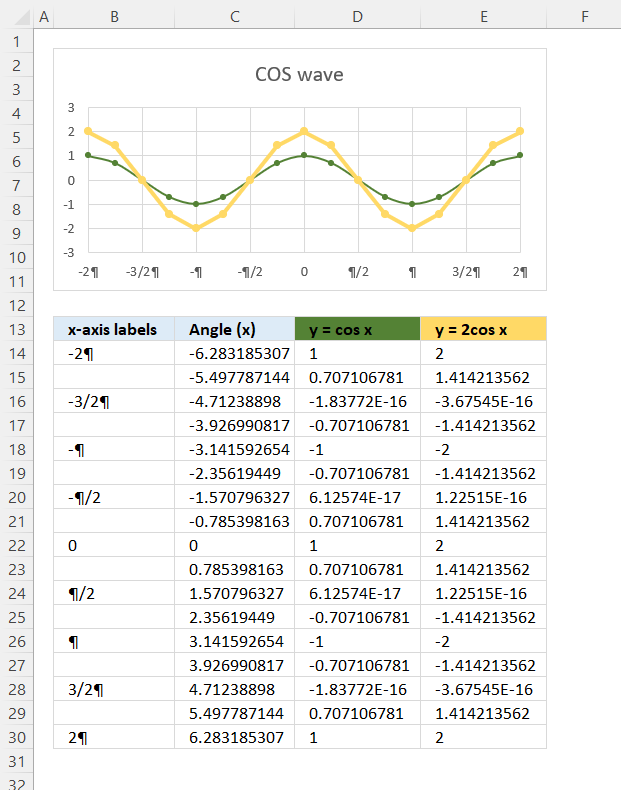The general form of the COSINE wave is y = A*COS(B*(x-C))+D

Constant A in the formula above changes the amplitude or height of the cosine wave. The image above shows two different cosine waves, a green and a yellow one.

The yellow cosine wave has an amplitude or height of 2 and the green one has an amplitude of 1.

Formula in cell D14:

=COS(B3)

Formula in cell E14:

=2*COS(B3)

## 7. How to change the cosine wave period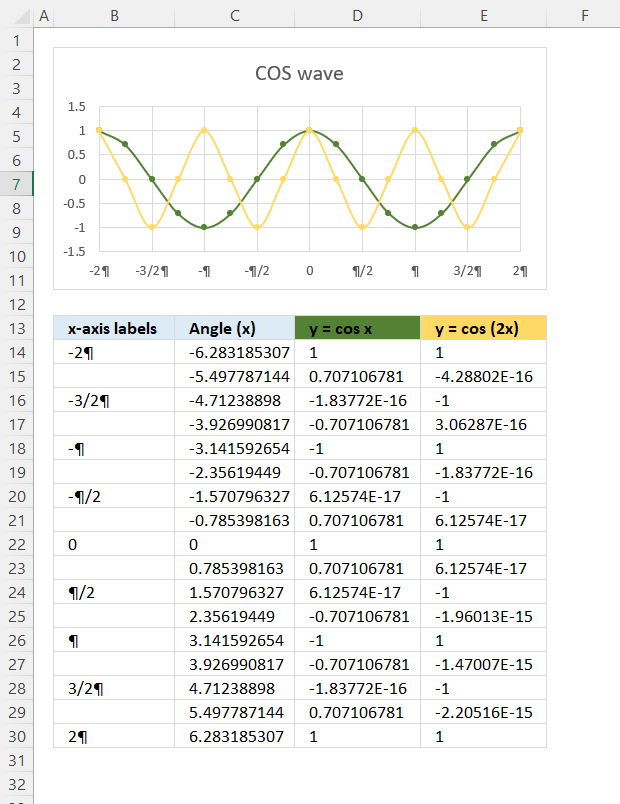The general form of the cosine wave is y = A*COS(B*(x-C))+D

Constant B changes the period, the chart above shows two cosine waves. The green cosine wave completes a cycle in 2π or 360°, however, the yellow cosine wave completes two cycles in 2π or 360°.

Formula in cell C3:

=COS(B3)

Formula in cell D3:

=COS(2*B3)

## 8. How to change the cosine wave midline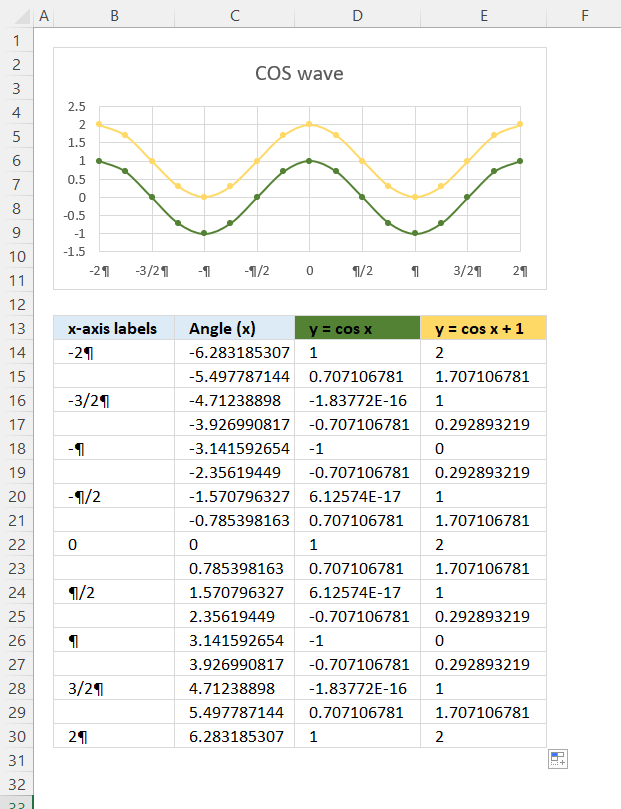The general form of the Sin wave is y = A*Sin(B*(x-C))+D

Constant D lets you change the midline which is the centerline in which the cosine wave oscillates back and forth. The midline is horizontal and is right in between the minimum and maximum cosine function values.

Formula in cell D14:

=COS(B3)

The green cosine wave in column D has a maximum value of 1 and a minimum value of -1, the midline is 0 (zero).

Formula in cell E14:

=COS(B3)+1

The yellow cosine wave in column E has a maximum value of 2 and a minimum value of 0, the midline is 1 (zero).

## 9. How to use the COS function with a right or right-angled triangle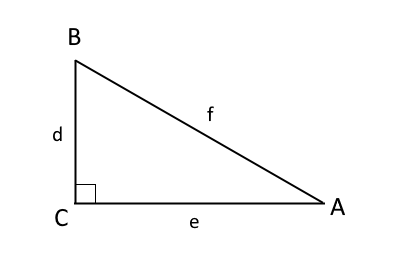A, B and C are angles, C is 90°, shown in the image above. A + B + C = 180°. One of the angles must be 90° (right angle triangle), in the example above C is 90°.

d, e, and f are lengths of the sides of the triangle. Side d and e are perpendicular to each other, meaning the angle between them are 90°.

COSINE A = e/f

COSINE A is equal to the ratio between sides e and f, this is only true if the triangle is a right-angled triangle.